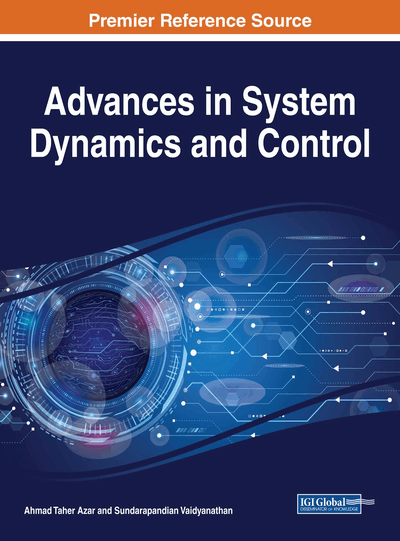# Dynamics Analysis and Synchronization in Relay Coupled Fractional Order Colpitts Oscillators

Kammogne Soup Tewa Alain, Kengne Romanic, Ahmad Taher Azar, Sundarapandian Vaidyanathan, Fotsin Hilaire Bertrand, Ngo Mouelas Adèle
DOI: 10.4018/978-1-5225-4077-9.ch011
OnDemand:
(Individual Chapters)
Available
\$29.50
No Current Special Offers

## Abstract

In this chapter, the dynamics of a particular topology of Colpitts oscillator with fractional order dynamics is presented. The first part is devoted to the dynamics of the model using standard nonlinear analysis techniques including time series, bifurcation diagrams, phase space trajectories plots, and Lyapunov exponents. One of the major results of this innovative work is the numerical finding of a parameter region in which the fractional order Colpitts oscillator's circuit experiences multiple attractors' behavior. This phenomenon was not reported previously in the Colpitts circuit (despite the huge amount of related research works) and thus represents an enriching contribution to the understanding of the dynamics of Chua's oscillator. The second part of this chapter deals with the synchronization of fractional order system. Based on fractional-order Lyapunov stability theory, this chapter provides a novel method to achieve generalized and phase synchronization of two and network fractional-order chaotic Colpitts oscillators, respectively.
Chapter Preview
Top

## Introduction

Fractional calculus is a mathematical topic that deals with derivatives and integration of arbitrary order (Miller & Ross, 1993). The commonly used definitions are those of Riemann–Liouville and Grünwald-Letnikov (Herrmann, 2011; Gorenflo & Mainardi, 1997; Samko et al., 1993). An important history of fractional calculus can be found in Machado et al. (2011). Although the subject is as old as the conventional calculus, it did not attract enough attention until recent decades. Nowadays, it has been known that many systems in interdisciplinary fields, such as viscoelastic materials, (Bagley & Calico 1991), electrode electrolyte polarization (Ichise et al., 1971), thermoelectric systems (Ezzat & El-Karamany 2012), finance systems (Laskin, 2000), dielectric polarization (Sun et al., 1984), Control systems (Azar et al., 2017a,b,c,d; Meghni et al., 2017a,b,c; Boulkroune et al, 2016a,b; Azar & Zhu, 2015), synchronization (Wang et al., 2017; Soliman et al., 2017; Tolba et al., 2017 ; Singh et al., 2017 ; Grassi et al., 2017), boundary layer effects in ducts, Electromagnetic waves, signal processing, and bio-engineering, can be elegantly described by fractional differential derivatives. The fractional calculus provides effective tools for the description of the memory and hereditary properties of various materials and processes.

The application of fractional-order calculus to nonlinear physics and its applications is only a recent focus of interest (Azar et al., 2017a; Ouannas et al., 2017a,b,c,d,e,i; Pham et al., 2017a; Ouannas et al., 2016a,b). Chaotic systems are deterministic and nonlinear dynamical systems which are exponentially sensitive to initial conditions (Yang et al., 2009; Lamamra et al., 2017; Vaidyanathan & Azar, 2017a,b,c; Vaidyanathan & Azar, 2016a,b,c,d,e,f). It’s well-known that chaos is ubiquitous in most nonlinear systems which have some additional properties, such as chaotic attractors and fractal motion (Azar et al., 2017b; Azar, & Vaidyanathan, 2015a,b,c; Azar, & Vaidyanathan, 2016). Moreover, many studies have demonstrated that many fractional-order differential systems, such as the fractional-order Lorenz system (Grigorenko & Grigorenko 2003), fractional order Duffling system (Ge & Ou, 2007), fractional-order Chua’s system (Hartley, et al., 1995), and fractional-order Chen’s system (Deng & Li, 2005) exhibit chaotic behaviors. Recently, Li et al. (2010) have studied the definition of Lyapunov exponents of fractional differential systems, which are often used to detect chaos in fractional differential systems. On the other hand, both frequency-domain methods and time-domain methods are used for computing the fractional differential systems (Li & Peng, 2004; Li et al., 2011). It was noted that using the frequency-domain approximation methods can conceal chaotic behaviour for a chaotic fractional order system or display chaos for a non-chaotic one (Tavazoei & Haeri, 2007). In comparison, the time-domain approximation method provides more effective numerical simulations to recognize chaos in fractional differential systems (Li et al., 2010).

## Complete Chapter List

Search this Book:
Reset